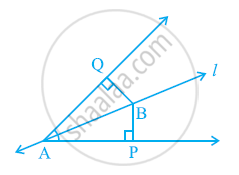# Line l is the bisector of an angle ∠A and B is any point on l. BP and BQ are perpendiculars from B to the arms of ∠A (see the given figure). Show that:- (i) ΔAPB ≅ ΔAQB (ii) BP = BQ or B is equidistant from the arms of ∠A. - Mathematics

Line l is the bisector of an angle ∠A and B is any point on l. BP and BQ are perpendiculars from B to the arms of ∠A (see the given figure). Show that:-

(i) ΔAPB ≅ ΔAQB

(ii) BP = BQ or B is equidistant from the arms of ∠A.#### Solution

In ΔAPB and ΔAQB,

∠APB = ∠AQB (Each 90º)

∠PAB = ∠QAB (l is the angle bisector of ∠A)

AB = AB (Common)

∴ ΔAPB ≅ ΔAQB (By AAS congruence rule)

∴ BP = BQ (By CPCT)

Or, it can be said that B is equidistant from the arms of ∠A.

Concept: Criteria for Congruence of Triangles
Is there an error in this question or solution?
Chapter 7: Triangles - Exercise 7.1 [Page 119]

#### APPEARS IN

NCERT Class 9 Maths
Chapter 7 Triangles
Exercise 7.1 | Q 5 | Page 119
Share